# SPSS Statistics

View Only

## Visualizing multi-level data using ellipses

####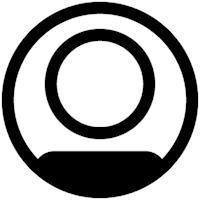By Archive User posted Tue March 04, 2014 10:20 AM

After reading Elliptical Insights: Understanding Statistical Methods through Elliptical Geometry (Friendly, Monette & Fox 2013) I was interested in trying ellipses out for viz. multi-level data. Note there is an add-on utility for SPSS to draw ellipses in R graphics (ScatterWEllipse.spd), but I wanted to give it a try in SPSS graphics.

So I've made two SPSS macros. The first, `!CorrEll`, takes two variables and returns a set of data that can be used by the second macro, `!Ellipse`, to draw data ellipses based on the eigenvectors and eigenvalues of those 2 by 2 covariance matrices by group. In this example I will be using the `popular2.sav` data available from Joop Hox's Multilevel Analysis book. The code can be downloaded from here to follow along.

So first lets define the `FILE HANDLE` where the data and scripts are located. Then we can read in the `popular2.sav` data. I only know very little about the data - but it is students nested within classrooms (pretty close to around 20 students in 100 classes), and it appears focused on student evaluations of teachers.

``FILE HANDLE Mac / name = "!Location For Your Data!".INSERT FILE = "MacMACRO_CorrEll.sps".INSERT FILE = "MacMACRO_EllipseSPSS.sps".GET FILE = "Macpopular2.sav".DATASET NAME popular2.``

Now we can call the first macro, `!CorrEll` for the two variables `extrav` (a measure of the teachers extroversion) and `popular` (there are two popular measures in here, and I am unsure what the difference between them are - this is the "sociometry" popular variable though). This will return a dataset with the means, variances and covariances for those two variables split by the group variable `class`. It will also return the major and minor diameters based on the square root of the eigenvalues of that 2 by 2 matrix, and then the ellipse is rotated according to direction of the covariance.

``!CorrEll X = extrav Y = popular Group = class.``

This returns a dataset named `CorrEll` as the active dataset, with which we can then draw the coordinate geometry for our ellipses using the `!Ellipse` macro.

``!Ellipse X = Meanextrav Y = Meanpopular Major = Major Minor = Minor Angle = AngDeg Steps = 100.``

The `Steps` parameter defines the coordinates around the circle that are drawn. So more steps means a more precise drawing (but also more datapoints to draw). This makes a new dataset called `Ellipse` as the active dataset, and based on this we can draw those ellipses in SPSS using the `path` element with the `split` modifier so the ellipses aren't drawn in one long pen stroke. Also note the ellipses are not closed circles (that is the first point does not meet the last point) so I use the `closed()` option when drawing the paths.

``FORMATS X Y Id (F3.0).GGRAPH  /GRAPHDATASET NAME="graphdataset" DATASET = 'Ellipse' VARIABLES=X Y Id  REPORTMISSING=NO  /GRAPHSPEC SOURCE=INLINE.BEGIN GPL SOURCE: s=userSource(id("graphdataset")) DATA: X=col(source(s), name("X")) DATA: Y=col(source(s), name("Y")) DATA: Id=col(source(s), name("Id"), unit.category()) GUIDE: axis(dim(1), label("Extraversion")) GUIDE: axis(dim(2), label("Popular")) ELEMENT: path(position(X*Y), split(Id), closed())END GPL.``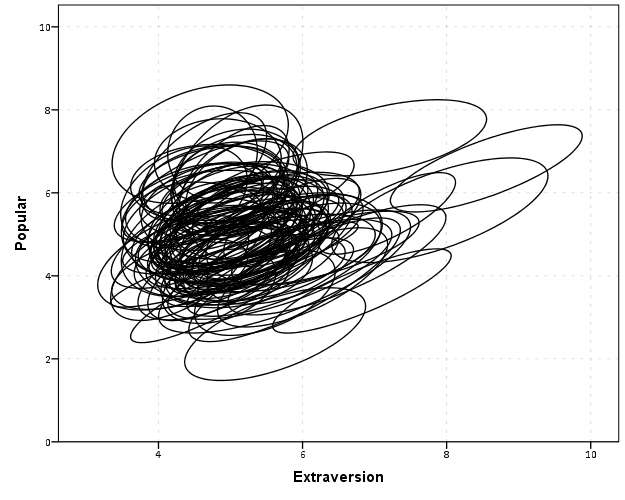With 100 groups this is a pretty good test of the efficacy of the display. While many multi-level modelling strategies will have fewer groups, if the technique can not scale to at least 100 groups it would be a tough sell. So above is a bit of an overplotted mess, but here I actually draw the polygons with a light grey fill and use a heavy amount of transparency in both the fill and the exterior line. To draw the ellipses I use the `polygon` element and connect the points using the `link.hull` statement. The `link.hull` modifier draws the convex hull of the set of points, which of course an ellipse is convex.

``GGRAPH  /GRAPHDATASET NAME="graphdataset" DATASET = 'Ellipse' VARIABLES=X Y Id  REPORTMISSING=NO  /GRAPHSPEC SOURCE=INLINE.BEGIN GPL SOURCE: s=userSource(id("graphdataset")) DATA: X=col(source(s), name("X")) DATA: Y=col(source(s), name("Y")) DATA: Id=col(source(s), name("Id"), unit.category()) GUIDE: axis(dim(1), label("Extraversion")) GUIDE: axis(dim(2), label("Popular")) ELEMENT: polygon(position(link.hull(X*Y)), split(Id), color.interior(color.grey), transparency.interior(transparency."0.8"),          transparency.exterior(transparency."0.5"))END GPL.``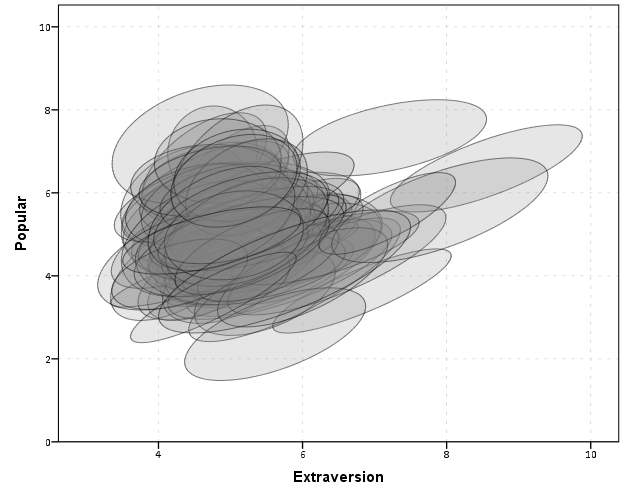I thought that using a fill might make the plot even more busy, but that doesn't appear to be the case. Using heavy transparency helps a great deal. Now what can we exactly learn from these plots?

First, you can assess the distribution of the effect of extroversion on popularity by class. In particular for multi-level models we can assess whether we can to include random intercepts and/or random slopes. In this case the variance of the extroversion slope looks very small to me, so it may be reasonable to not let its slope vary by class. Random intercepts for classes though seem reasonable.

Other things you can assess from the plot are if there are any outlying groups, either in coordinates on the x or y axis, or in the direction of the ellipse. Even in a busy - overplotted data display like this we see that the covariances are all basically in the same positive direction, and if one were strongly negative it would stick out. You can also make some judgements about the between group and within group variances for each variable. Although any one of these items may be better suited for another plot (e.g. you could actually plot a histogram of the slopes estimated for each group) the ellipses are a high data density display that may reveal many characteristics of the data at once.

A few other interesting things that are possible to note from a plot like this are aggregation bias and interaction effects. For aggregation bias, if the direction of the orientation of the ellipses are in the opposite direction of the point cloud of the means, it provides evidence that the correlation for the aggregate data is in the opposite direction as the correlation for the micro level data.

For interaction effects, if you see any non-random pattern in the slopes it would suggest an interaction between extroversion and some other factor. The most common one is that slopes with larger intercepts tend to be flatter, and most multi-level software defaults to allow the intercepts and slopes to be correlated when they are estimated. I was particularly interested in this here, as the popularity score is bounded at 10. So I really expected that to have a limiting effect on the extroversion slope, but that doesn't appear to be the case here.

So unfortunately none of the cool viz. things I mention (outliers, aggregation bias or interaction effects) really appear to occur in this plot. The bright side is it appears to be a convenient set of data to fit a multi-level model too, and even the ceiling effect of the popularity measure do not appear to be an issue.

We can add in other data to the plot from either the original dataset or the `CorrEll` calculated dataset. Here is an example of grabbing data from the `CorrEll` dataset and labelling the ellipses with their group numbers. It is not very useful for the dense cloud, but for the outlying groups you can pretty easily see which label is associated with each ellipse.

``DATASET ACTIVATE CorrEll.FORMATS Meanpopular Meanextrav class (F3.0).DATASET ACTIVATE Ellipse.GGRAPH  /GRAPHDATASET NAME="graphdataset" DATASET = 'Ellipse' VARIABLES=X Y Id  /GRAPHDATASET NAME="Center" DATASET = 'CorrEll' VARIABLES=Meanpopular Meanextrav class  REPORTMISSING=NO  /GRAPHSPEC SOURCE=INLINE.BEGIN GPL SOURCE: s=userSource(id("graphdataset")) DATA: X=col(source(s), name("X")) DATA: Y=col(source(s), name("Y")) DATA: Id=col(source(s), name("Id"), unit.category()) SOURCE: c=userSource(id("Center")) DATA: CentY=col(source(c), name("Meanpopular")) DATA: CentX=col(source(c), name("Meanextrav")) DATA: class=col(source(c), name("class"), unit.category()) GUIDE: axis(dim(1), label("Extraversion")) GUIDE: axis(dim(2), label("Popular")) ELEMENT: polygon(position(link.hull(X*Y)), split(Id), color.interior(color.grey), transparency.interior(transparency."0.8"),          transparency.exterior(transparency."0.5")) ELEMENT: point(position(CentX*CentY), transparency.exterior(transparency."1"), label(class))END GPL.``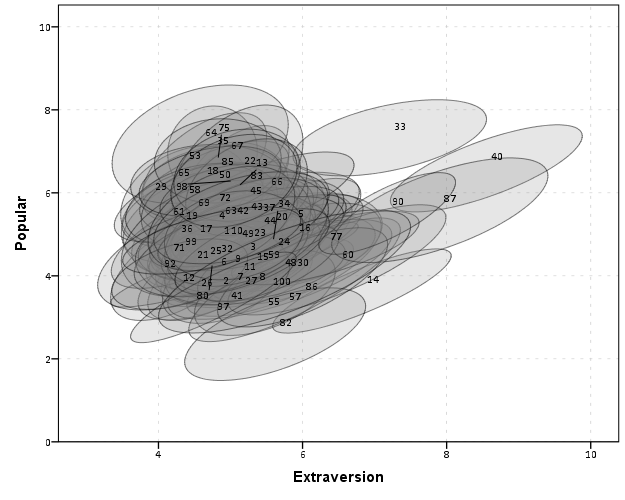Another piece of information we can add into the plot is to color the fill of the ellipses using some alternative variable. Here I color the fill of the ellipse according to teacher experience with a green to purple continuous color ramp. SPSS uses some type of interpolation through some color space, and the default is the dreaded blue to red rainbow color ramp. With some experimentation I discovered the green to purple color ramp is aesthetically pleasing (I figured the diverging color ramps from colorbrewer would be as good a place to start as any). I use a diverging ramp as I want a higher amount of discrimination for exploratory graphics like this. Using a sequential ramp ends up muting one end of the spectrum, which I don't really want in this circumstance.

``DATASET ACTIVATE popular2.DATASET DECLARE TeachExp.AGGREGATE OUTFILE='TeachExp'  /BREAK=Class  /TeachExp=FIRST(texp).DATASET ACTIVATE Ellipse.MATCH FILES FILE = *  /TABLE = 'TeachExp'  /RENAME (Class = Id)  /BY Id.FORMATS TeachExp (F2.0).*Now making plot with teacher experience colored.DATASET ACTIVATE Ellipse.GGRAPH  /GRAPHDATASET NAME="graphdataset" DATASET = 'Ellipse' VARIABLES=X Y Id TeachExp  REPORTMISSING=NO  /GRAPHSPEC SOURCE=INLINE.BEGIN GPL SOURCE: s=userSource(id("graphdataset")) DATA: X=col(source(s), name("X")) DATA: Y=col(source(s), name("Y")) DATA: TeachExp=col(source(s), name("TeachExp")) DATA: Id=col(source(s), name("Id"), unit.category()) GUIDE: axis(dim(1), label("Extraversion")) GUIDE: axis(dim(2), label("Popular")) GUIDE: legend(aesthetic(aesthetic.color.interior), label("Teacher Experience")) SCALE: linear(aesthetic(aesthetic.color.interior), aestheticMinimum(color.green), aestheticMaximum(color.purple)) ELEMENT: polygon(position(link.hull(X*Y)), split(Id), color.interior(TeachExp), transparency.interior(transparency."0.7"),          transparency.exterior(transparency."0.5"))END GPL.``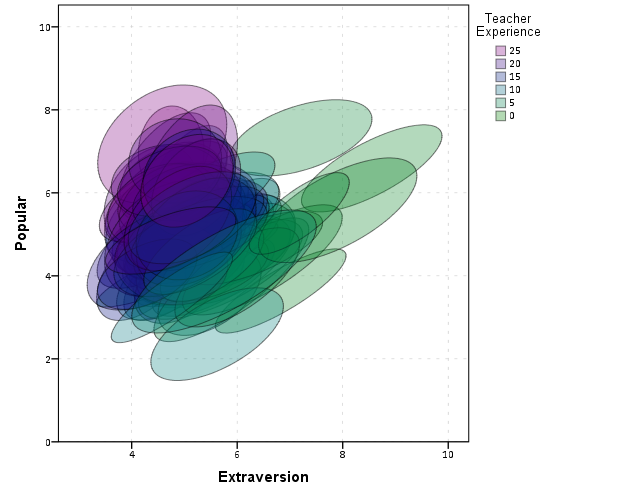Again I use a heavy amount of transparency and it produces what I think is a very nice looking plot. From this we can deduce that there is a clear relationship between extroversion and teacher experience, younger teachers tend to be more extroverted. We can also see that teacher experience explains some of the differences in means not explained by extroversion. That is some of the teachers with higher mean popular scores but lower extroversion scores are more experienced. This suggests the effects of teacher experience and extroversion are additive in the model predicting popularity.

You could of course color the ellipse with other variables as well. Because these are data ellipses and not confidence ellipses, you could make ellipses with fewer observations more transparent to illustrate that those estimates are less certain. Here the classrooms are all very similar size, so the error in the estimates is basically constant for all of the groups in this example.

The current code calculates the ellipses based on the eigenvectors and eigenvalues of the covariance matrix, but I may change this in the future to calculate them based on the Cholesky decomposition. If you read the Friendly paper most of the notation is written in terms of the Cholesky decomposition, and this would allow one to estimate confidence ellipses as well as the data ellipses here. So you could draw an ellipse that shows a confidence interval as opposed to the ellipses here that are just one possible level curve through the bivariate normal estimate.

Another thing I noticed the other day in the bowels of the SPSS chart template was that the xml defined glyphs had a rotation and an aspect parameter, so you could actually make a set of ellipse glyphs (although to cycle through them in SPSS charts is a pain). That makes me think that rotation and aspect should be mappable in the grammar of graphics, but I am unfamiliar with any statistical packages that allow you to easily manipulate figures in plots by specifying either the rotation of a glyph or the aspect of the glyph.

Let me know if there are any other good examples of using ellipses to visualize multi-level data.

#datavisualization
#ellipses
#MACRO
#multi-level
#SPSS
#SPSSStatistics
#Visualization
4 views